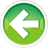# O.1 — Bit flags and bit manipulation via std::bitset

On modern computer architectures, the smallest addressable unit of memory is a byte. Since all objects need to have unique memory addresses, this means objects must be at least one byte in size. For most variable types, this is fine. However, for Boolean values, this is a bit wasteful. Boolean types only have two states: true (1), or false (0). This set of states only requires one bit to store. However, if a variable must be at least a byte, and a byte is 8 bits, that means a Boolean is using 1 bit and leaving the other 7 unused.

In the majority of cases, this is fine -- we’re usually not so hard-up for memory that we need to care about 7 wasted bits (we’re better off optimizing for understandability and maintainability). However, in some storage-intensive cases, it can be useful to “pack” 8 individual Boolean values into a single byte for storage efficiency purposes.

Doing these things requires that we can manipulate objects at the bit level. Fortunately, C++ gives us tools to do precisely this. Modifying individual bits within an object is called bit manipulation.

Bit manipulation is also useful in encryption and compression algorithms.

Bit flags

Up to this point, we’ve used variables to hold single values:

However, instead of viewing objects as holding a single value, we can instead view them as a collection of individual bits. When individual bits of an object are used as Boolean values, the bits are called bit flags.

As an aside...

In computing, a flag is a value that acts as a signal for some function or process. Analogously, in real life, a mailbox flag is used to signal that there is something inside the mailbox, so the mailbox doesn’t have to be opened to check.

To define a set of bit flags, we’ll typically use an unsigned integer of the appropriate size (8 bits, 16 bits, 32 bits, etc… depending on how many flags we have), or std::bitset.

Best practice

Bit manipulation is one of the few times when you should unambiguously use unsigned integers (or std::bitset).

In this lesson, we’ll show how to do bit manipulation the easy way, via std::bitset. In the next set of lessons, we’ll explore how to do it the more difficult but versatile way.

Bit numbering and bit positions

Given a sequence of bits, we typically number the bits from right to left, starting with 0 (not 1). Each number denotes a bit position.

```76543210  Bit position
00000101  Bit sequence
```

Given the bit sequence 0000 0101, the bits that in position 0 and 2 have value 1, and the other bits have value 0.

Manipulating bits via std::bitset

In lesson 4.13 -- Literals we already showed how to use a std::bitset to print values in binary. However, this isn’t the only useful thing std::bitset can do.

std::bitset provides 4 key functions that are useful for doing bit manipulation:

• test() allows us to query whether a bit is a 0 or 1
• set() allows us to turn a bit on (this will do nothing if the bit is already on)
• reset() allows us to turn a bit off (this will do nothing if the bit is already off)
• flip() allows us to flip a bit value from a 0 to a 1 or vice versa

Each of these functions takes a bit-position argument indicating which bit position we want operated on.

Here’s an example:

This prints:

```All the bits: 00001101
Bit 3 has value: 1
Bit 4 has value: 0
```

What if we want to get or set multiple bits at once

std::bitset doesn’t make this easy. In order to do this, or if we want to use unsigned integer bit flags instead of std::bitset, we need to turn to more traditional methods. We’ll cover these in the next couple of lessons.O.2 -- Bitwise operatorsIndex5.x -- Chapter 5 comprehensive quiz

### 1 comment to O.1 — Bit flags and bit manipulation via std::bitset

•nascardriver